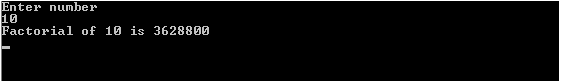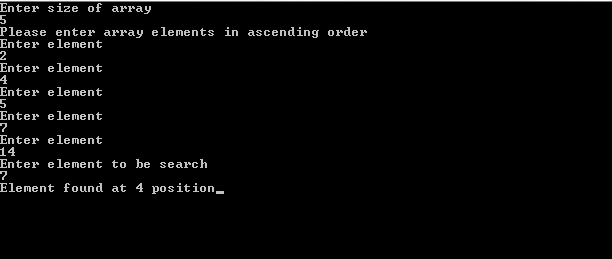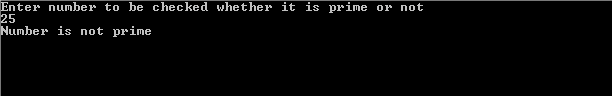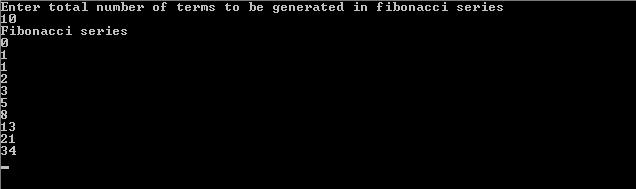# C Program Examples## Factorial Program in C

Factorial program in c using function and recursion with the output is given below. About Factorial The factorial of a non negative integer, n, is the product of all positive integers less than or equal to n. It is denoted by n!. Also, the value of 0! is 1. For example, 3!=3*2*1=6 Recursively, factorial can be defined as, n! =… Read more →## Binary Search Program in C

Binary search program in c using function and without using function is given below with the output. About Binary Search Binary search is a divide and conquer search algorithm used primarily to find out the position of a specified value within an array. It should be noted that, for binary search to operate on arrays, the array must be sorted… Read more →## Prime Number Program in C – C Program for Prime Number

Prime number program in c along with the output is given below. About Prime Numbers Any natural number like 1, 2, 3, etc. is called a prime or a prime number if it is greater than 1 and can only be divided by 1 and the number itself. For example, 2, 3, 5, 7, 11, 13, 17, 19, 23, 29,…,… Read more →## C Program for Fibonacci Series

C Program for Fibonacci Series using for loop, recursion and array along with the output is given below. About Fibonacci Series The Fibonacci series or Fibonacci numbers or Fibonacci sequence always consists of 0 and 1 as its first two numbers with each subsequent number in the series as the sum of the previous two numbers. Mathematically, the sequence Fn… Read more →